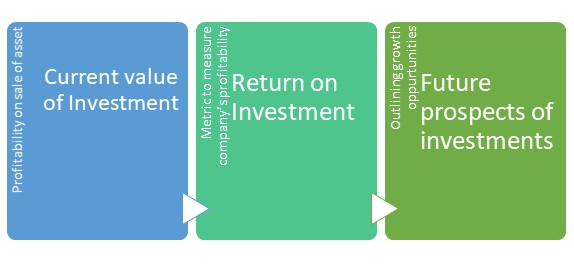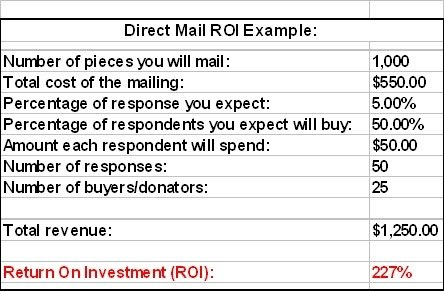# Return on Investment (ROI) Definition, Importance, Example, Types, Formula & Overview

Posted in Finance, Accounting and Economics Terms, Total Reads: 1908

## Definition: Return on Investment (ROI)

Return on investment or ROI, is the measure to evaluate the efficiency of an investment in a venture or multiple ventures. Return on Investment directly measures the return one gets or to simplify, the benefits one gets out of investing a certain sum in an opportunity. ROI is expressed in percentage terms, and is calculated as the benefit or return received by the total amount of investment as a ratio.

Return on investments for different investments can be compared because it is expressed in the ratio or percentage format. Therefore, comparison becomes easier. ROI is an effective measure which helps us decide whether we should make an investment in the first place or not. The Return on Investment formulae is used in investing and financing. There are other similar formulas also that measure the profitability of the organization- Return on Capital, Return on Equity, Return on assets and many others.

## Importance of Return on Investment (ROI)

Return on investment is used as a metric to compare the company’s profitability to the company’s efficiency in making investments. It is one of the most used profitability ratios because of its flexible use. Since it is expressed as a ratio or percentage different investments can be compared with each other. Return on investment must be very carefully and accurately measured because even a little change can lead to a massively incorrect analysis. It is very important to use the same inputs across all different investments to get appropriate measures of return on investments and to be able to have a successful comparison. As return on investment calculation does not take into account the time period of cash flows generated it is very essential to accurately estimate the rest of the inputs. Return on Investment tells us whether the investment is beneficial or not. If the ROI is positive it indicates the investment is worthwhile. Therefore, we see investments with higher ROI are much more beneficial than the ones with lower ROI. When compared with Net Present Value as a metric ROI gives relatively different results because it doesn’t take the time value of money into account.ROI is a measurement of profit earned from the investments made. Return on investment evaluates the earning power of the investment done in assets, which is measured as the ratio of profit less depreciation (net income) to the equity capital (average capital employed) in a project or company. It directly compares the timing and the magnitude of investment gains with the timing and magnitude of costs. Higher the Return on Investment, higher would be favorability of gains to cost.

When the Long- Term Return on Investment is lesser than the Cost of Capital. This puts the company in a situation where liquidating its assets and depositing the amount in the bank will put the company in a better off place. Such a situation indicates that the returns from the asset purchased is lower than the amount spend on attaining and maintenance of the asset. ROI is a very important metric for calculating the financial consequences. There are many metrics available, but the best known metric is the Return on Investment. It is becoming increasingly popular as it helps in evaluating projects, capital acquisitions, initiatives and programs. ROI also helps in evaluating the traditional financial investments in stocks.

## Formula of Return on Investment (ROI)

ROI = (Current Value of Investment - Cost of Investment) / Cost of Investment

The return is measure as the value remaining after the cost of investment is borne completely by the current value of the asset. The current value of investment refers to the proceeds obtained from the sale of the asset.

Return on investment can also be measure by the following method,

ROI = (Net Profit / Cost of Investment) x 100

## Types of Return on Investment (ROI)

1. To measure Rate of Earnings on the Total Capital Employed: Divide net income, taxes and interest by total liabilities

2. To measure Rate of Earnings of the Invested Capital: Divide income taxes and net income by fixed liability and proprietary equity.

3. To measure Rate of Earnings on stock and proprietary equity: Divide net income by total capital and reserves.

ROI is expressed in percentages; therefore the answer must be multiplied by 100.

## Examples of Return on Investment (ROI)

1. Consider a company sending direct mailers for B2B marketing:2. If an investor buys a house for \$100000 and sells it after five years and gets a net profit of \$20000 the ROI can be calculated as:

ROI = (20000/100000) *100 = 20%

The return on investment is 20% but the calculation may change if taxes are involved. This shows that the investment is profitable and the company’s efficiency in investment is good.

Hence, this concludes the definition of Return on Investment (ROI) along with its overview.

Browse the definition and meaning of more terms similar to Return on Investment (ROI). The Management Dictionary covers over 7000 business concepts from 6 categories. This definition and concept has been researched & authored by our Business Concepts Team members.

Search & Explore : Management Dictionary

Similar Definitions from same Category: